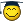# Mousedown problems

## Recommended Posts

EDIT: Problem solved, the mouse was going down while it was still over the guiI wrote some code that moves the mouse in a perfect circle. I thought it was pretty clever. But the mousedown part doesn't work. It works fine in a straight line, even multiple points in a straight line, but when it's in a scircle it's not working. Probably something simple I don't know about that I'm missing - maybe with the for... to loop?

```MouseDown("Primary")
\$dir = 1 ; down and to the right
For \$temp = 1 To 2
; This only draws half-circles - so we need to do it twice, and invert it the second time.
;~                          formula   x ^ 2 + y ^ 2 = r ^ 2
\$x = \$i
\$y = Sqrt((\$radius ^ 2) - (\$x ^ 2))
\$x = Round(\$x * \$dir) + \$Start_x
\$y = Round(\$y * \$dir) + \$Start_y
; MsgBox(4096, "Coords", "x=" & \$x & " y=" & \$y)
If \$close = True Then ExitLoop
MouseMove(\$x, \$y, \$speed)
Next
\$dir = -1 ; up and to the left
Next
MouseUp("primary")```
Edited by danielmohr91

## Create an account

Register a new account

• ### Recently Browsing   0 members

×

• Wiki

• Back

• #### Beta

• Git
• FAQ
×
• Create New...